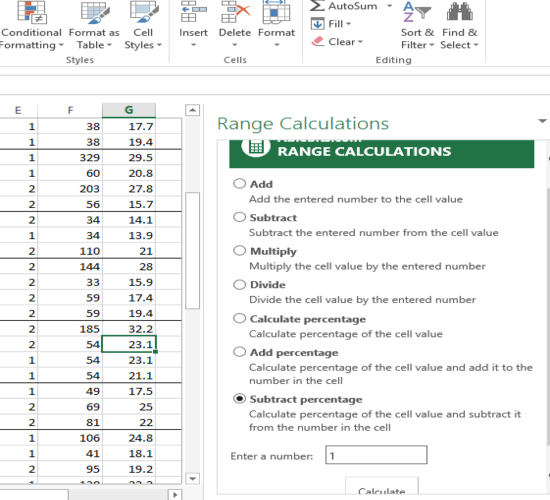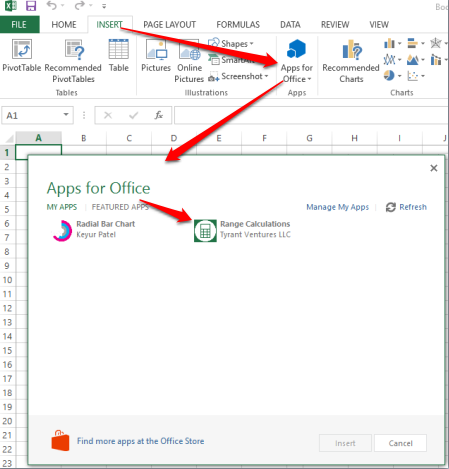Editor Ratings:
User Ratings:
[Total: 0 Average: 0]

Range Calculations is a free Excel add-in to automatically change the values of multiple cells of an Excel sheet using any number. Suppose you want to add a particular digit in multiple cells of an Excel sheet that includes pre-added values. Doing this manually won’t be a good idea because it is time consuming and also not convenient. In that case, this add-in will come in handy. All you need to do is select cells, a number (5, 10, 15.3, or anything) and perform addition in all those values in a single click.

Apart from addition, you can also divide, multiply, and subtract the values of cells in bulk using a preferred number. In the recording below, you can see exactly what I mentioned.## Using This Free Excel Add-In To Change The Values of Multiple Cells of An Excel Sheet:

Step 2: Now open Excel sheet→ click on INSERT→ Apps for Office. It will open a pop-up using which you can select and insert this add-in. Not to mention that you must be signed in with the same Office account to access the add-in.Step 3: After pressing the Insert button, the right sidebar of this add-in will be in front of you.Step 4: The sidebar contains multiple options. All you need to do is select an option, select cells where changes are required, enter a number that will be used for changes, and click Calculate button. It will immediately update values of all those selected cells. Available options are:

• Add: To add a number to all the selected cells. For example, if you have selected three cells which contain 4, 5, 6 as values and you add 2 as your number, then values of all those cells will be set to 6, 7, and 8.
• Subtract: To subtract values with a particular number, this option is used.
• Multiply:  To multiply and update values with a custom number, you can use this option.
• Divide: Divide and update all the selected values with a specific number.
• Calculate Percentage: If you want to calculate the 1 percent of the values of multiple cells, you can use this option. The output will show the new values that will come by calculating the percentage. Original values are replaced with percentage values.
• Add Percentage: This option works similar to the above option. The only thing is that instead of replacing the original values, it keeps the original values, plus add percentage in those values. For example, if a value is 2 and you calculated 1 percent (0.02) of that value, then it will update the value as 2.02.
• Subtract Percentage: Using this option, the original values are subtracted with the output percentage and final value is stored in those cells.

This is all you can do using this add-in.

## The Verdict:

Range Calculations Excel add-in is surely a good option when you need to bulk change values of multiple cells of your Excel sheet. Only a few mouse clicks and number is needed to change and update values of cells.